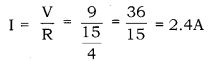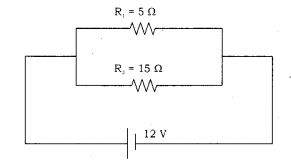# Two resistors with resistances 5Ω and 15Ω are to be connected to a battery of emf 9V so as to obtain: (i) minimum current

Two resistors with resistances 5Ω and 15Ω are to be connected to a battery of emf 9V so as to obtain:
(i) minimum current
(ii) maximum current.
(a) Show with the help of a diagram how you would connect the resistances in each case.
(b) Calculate the strength of the total current in the circuit in each of the two cases.

(a)

1. For minimum current flow: In order to obtain the minimum current flowing through the circuit, the resistors must be connected in series with each other.
2. For maximum current flow: In order to obtain the maximum current flowing through the circuit, the resistors must be connected in parallel with each other.

(b)

1. For minimum current flow: Two resistors of resistances R1 and R2 are connected in series as:
The equivalent resistance of the circuit is given as:
R = R1 + R2 = 5 + 15 = 20Ω
According to Ohm’s Law:
V = IR
Where,
V = emf of the battery = 9V
I = Total current flowing through the circuit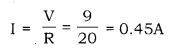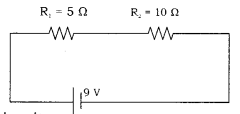1. For maximum current flow: Two resistors of resistances R1 and R2 are connected in parallel as:

The equivalent resistance of the circuit is given as: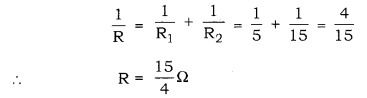According to Ohm’s law
V = IR
V = emf of the battery =9v
I = Total current flowing through the circuit